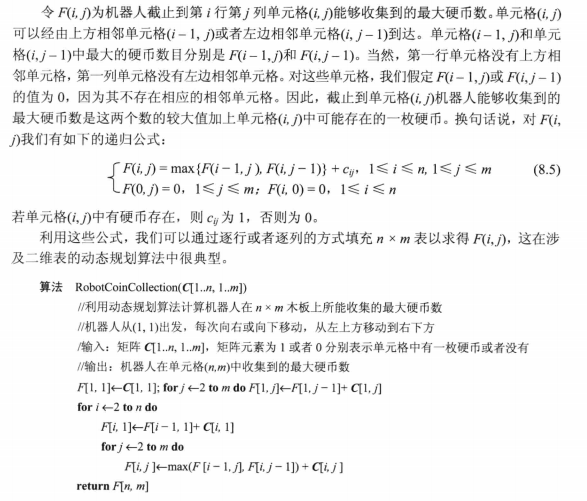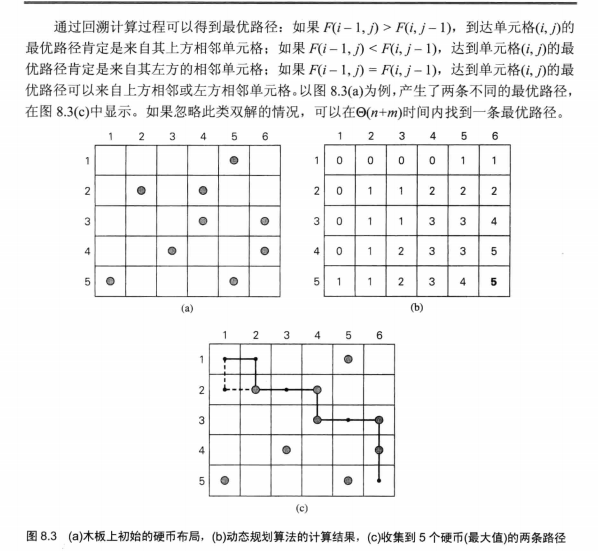0%

## 币值最大化问题

$$F\left( n \right) = \max \left\{ {{c_n} + F\left( {n - 2} \right),F\left( {n - 1} \right)} \right\},n > 1$$ $$F\left( 0 \right) = 0,F\left( 1 \right) = {c_1}$$

## 找零问题

$$\left\{ \begin{gathered} F(n) = \mathop {\min }\limits_{j:n \geqslant {d_j}} \{ F(n - {d_j})\} + 1,n > 0 \hfill \\ F(0) = 0 \hfill \\ \end{gathered} \right.$$

## 硬币收集问题